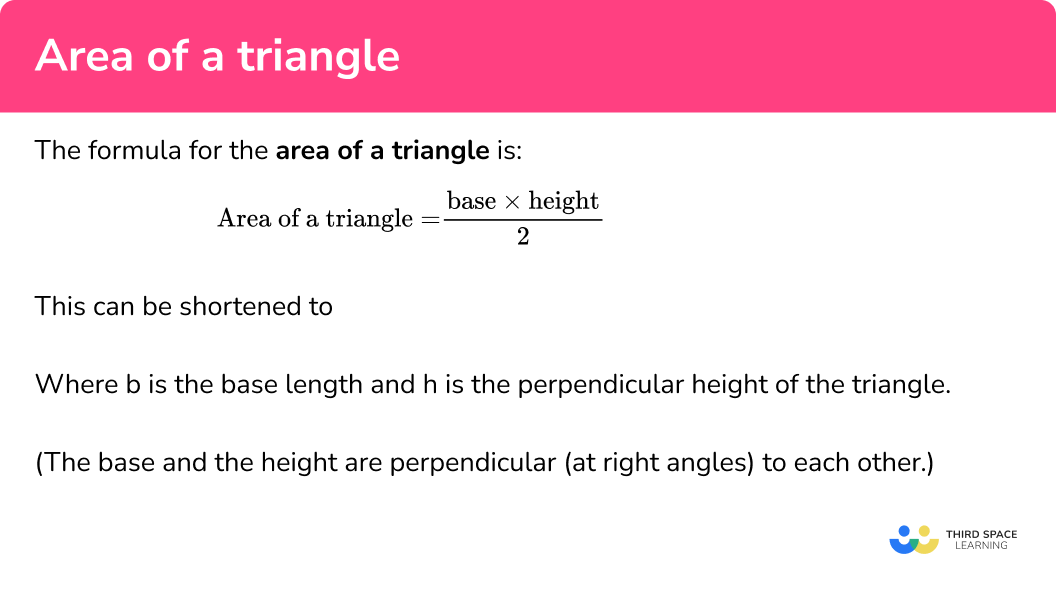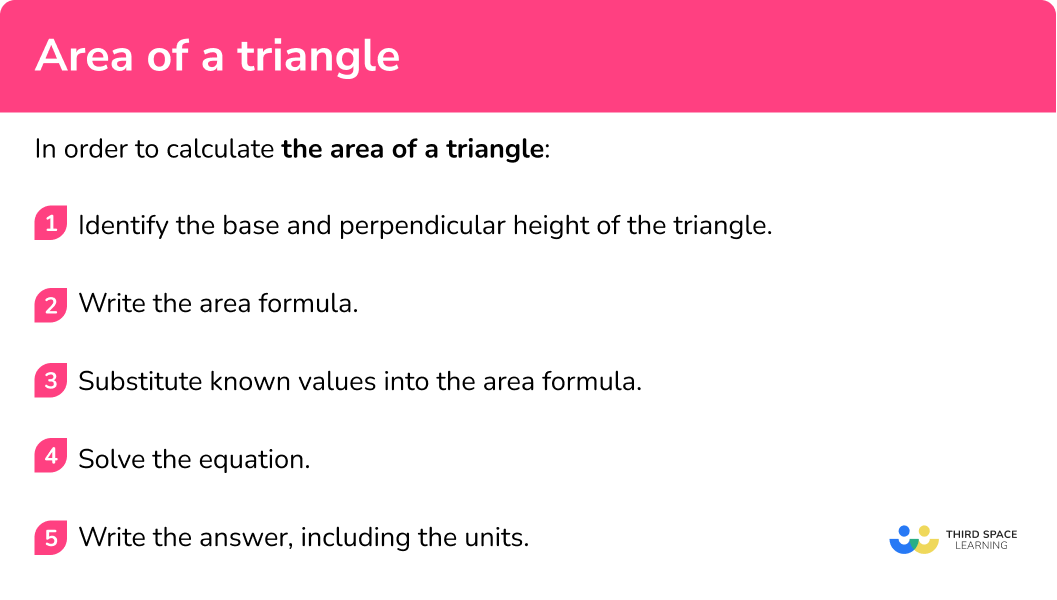GCSE Maths Geometry and Measure Area

Area Of A Triangle

# Area Of A Triangle

Here we will learn about the area of a triangle, including how to find the area of a triangle with given dimensions.

There are also area of a triangle worksheets based on Edexcel, AQA and OCR exam questions, along with further guidance on where to go next if you’re still stuck.

## What is the area of a triangle?

The area of a triangle is the amount of enclosed two-dimensional space that three connected straight lines take up.

In order to derive the area of a triangle, we start with the area of a rectangle.

The area of the rectangle below would be calculated by multiplying the base by the height ( b \times h ). This is usually pronounced as  “base times height”.

We can split a rectangle into two smaller rectangles by drawing a perpendicular line through any two parallel sides of the rectangle.

Splitting these two rectangles in half by joining the diagonals together, we can create two pairs of congruent (identical) right-angled triangles.

The area of the rectangle is now halved to get the area of the triangle, so the area of the triangle is exactly half the area of the rectangle, when we know the base and the perpendicular height of the triangle.

### What is the area of a triangle?### Area of a right angled triangle formula

The formula for the area of a triangle is:

\text{Area of a triangle}=\frac{\text{base }\times \text{ height}}{2}

This can be shortened to

A=\frac{1}{2}bh

where b is the base length and h is the perpendicular height of the triangle.

(Perpendicular means that the base and the height are at right angles to each other.)

Your final answer must be given in square units, e.g. cm^2, \ m^2, or mm^2 .

Higher – There is an alternative method to find the area of a triangle which involves sine, two sides and the included angle. This is part of trigonometry.

\text{Area of a triangle}=\frac{1}{2}ab\sin{C}

Step-by-step guide: Area of a triangle ( \frac{1}{2}ab \sin C )

On this page we’ll look at the area of scalene triangles. A scalene triangle is a triangle where all of the sides and all of the angles are different.

This means that a scalene triangle can contain three acute angles, a right-angle, or one obtuse angle (and two acute angles).

Remember: All of the angles within any triangle must add up to 180^{\circ} .

### Labelling triangles

The triangle below has the three sides labelled a, b, and c, and the three vertices labelled A, B, and C. The side a opposes the vertex A and so on.

You should also be familiar with the notation that this triangle is called triangle ABC .

Each side could also be labelled using the capital letters that denote the start and end point of the line. For example, the side c is also known as the side AB , the side a can be referred to as side BC , and side b can be expressed as side AC .

## How to calculate the area of a triangle

In order to calculate the area of a triangle:

1. Identify the base and perpendicular height of the triangle.
2. Write the area formula.
3. Substitute known values into the area formula.
4. Solve the equation.
5. Write the answer, including the units.

### How to calculate the area of a triangle## Related lessons on area

Area of a triangle is part of our series of lessons to support revision on area. You may find it helpful to start with the main area lesson for a summary of what to expect, or use the step by step guides below for further detail on individual topics. Other lessons in this series include:

## Area of a triangle examples

### Example 1: right-angled scalene triangle

Calculate the area of the right-angled triangle ABC . Write your answer in square centimetres.

1. Identify the base and perpendicular height of the triangle.

The sides AC and BC are perpendicular to each other (the angle at C is a right-angle) and so we can state the values for the base and the perpendicular height of the triangle to be:

The base: b=3.2 \ cm

The perpendicular height: h=5.3 \ cm

2Write the area formula.

A=\frac{1}{2}bh

3Substitute known values into the area formula.

\begin{aligned} A &=\frac{1}{2}bh \\\\ &= \frac{1}{2}(3.2)(5.3) \end{aligned}

4Solve the equation.

Work out the value for A by solving the equation:

\begin{aligned} A &=\frac{1}{2}bh \\\\ &= \frac{1}{2}(3.2)(5.3) \\\\ &=\frac{1}{2}\times 3.2\times 5.3\\\\ &=8.48 \end{aligned}

5Write the answer, including the units.

A=8.48\text{ cm}^2

### Example 2: obtuse scalene triangle

For triangle ABC , the angle at C is an obtuse angle, the length AC=12cm , and the height of the triangle is 9cm . Calculate the area of triangle ABC.

Identify the base and perpendicular height of the triangle.

Write the area formula.

Substitute known values into the area formula.

Solve the equation.

Write the answer, including the units.

### Example 3: acute scalene triangle – mixed units

Calculate the area of triangle ABC below. Write your answer in square metres.

Identify the base and perpendicular height of the triangle.

Write the area formula.

Substitute known values into the area formula.

Solve the equation.

Write the answer, including the units.

### Example 4: obtuse scalene triangle – too much information

PQR is an obtuse scalene triangle. The point S lies on the line PQ such that angle PSR=90^{\circ} .

Calculate the area of the triangle PQR below, correct to 2 decimal places.

Identify the base and perpendicular height of the triangle.

Write the area formula.

Substitute known values into the area formula.

Solve the equation.

Write the answer, including the units.

### Example 5: compound shape

A rectangle and a triangle are placed together to make a compound shape ABCDE .

Calculate the area of the compound shape to 1 decimal places.

Identify the base and perpendicular height of the triangle.

Write the area formula.

Substitute known values into the area formula.

Solve the equation.

Write the answer, including the units.

## How to find a missing side length given the area

Sometimes a question might give you the area and ask you to work out the missing length. In order to do this you must rearrange the formula to make the missing value the subject, and then solve for this letter.

In order to find a missing length given the area:

1. Write the area formula.
2. Substitute the known values into the area formula.
3. Rearrange the equation and solve.
4. Write the answer, including the units.

## Missing side length example

### Example 6: calculating the base length given the area

The area of a triangle is 45 \ cm^2 . The height of the triangle is 10 \ cm. Find the length of the base of the triangle.

Write the area formula

Substitute the known values into the area formula

Rearrange the equation and solve.

Write the answer, including the units.

### Common misconceptions

• Identifying the correct information to use

The base and the height must be at a right angle to each other. They must be perpendicular. You may be given additional sides of a triangle that are not needed. You may also need to rotate the triangle around so that you can identify the base and height more easily.

• Units

It is common to forget the units for area in the final answer. When calculating area, your answer must always have units squared (or square units).

### Practice area of a triangle questions

1. Calculate the area of the triangle:8\text{ cm}^27.5\text{ cm}^215\text{ cm}^216\text{ cm}^2The base and the perpendicular height are 5 \ cm and 3 \ cm respectively, so the calculation we need to do is:

\begin{aligned} A&=\frac{1}{2}bh \\\\ &=\frac{1}{2}(5)(3) \\\\ &=\frac{1}{2}\times 5\times 3 \\\\ &=7.5 \end{aligned}

The units will be cm^2.

2. Find the area of the triangle. Give your answer in cm^2:126\text{ cm}^2252\text{ cm}^212.6\text{ cm}^225.2\text{ cm}^2The base is 63 \ mm which needs to be converted to 6.3 \ cm. The height is 4 \ cm. The calculation we need to do is:

\begin{aligned} A&=\frac{1}{2}bh \\\\ &=\frac{1}{2}(6.3)(4) \\\\ &=\frac{1}{2}\times 6.3\times 4 \\\\ &=12.6 \end{aligned}

The units will be cm^2.

3. Find the area of the triangle:24\text{ cm}^221\text{ cm}^212\text{ cm}^210.5\text{ cm}^2It may be easier to see the base and height if you turn the triangle. The base and the height are 3 \ cm and 7 \ cm. The 8 \ cm is not needed. So the calculation we need to do is:

\begin{aligned} A&=\frac{1}{2}bh \\\\ &=\frac{1}{2}(3)(7) \\\\ &=\frac{1}{2}\times 3\times 7 \\\\ &=10.5 \end{aligned}

The units will be cm^2.

4. Triangle ABC is an obtuse scalene triangle. The angle at A=120^{\circ} . Calculate the area of the triangle. Give your answer in m^2 :13.8 \text{ m}^213 \ 800 \text{ cm}^26.9\text{ m}^269 \ 000\text{ cm}^2The base is 460 \ cm which needs to be converted to 4.6 \ m. The height is 3 \ m. The calculation we need to do is:

\begin{aligned} A&=\frac{1}{2}bh \\\\ &=\frac{1}{2}(4.6)(3) \\\\ &=\frac{1}{2}\times 4.6 \times 3 \\\\ &=6.9 \end{aligned}

The units will be m^2.

5. Find the area of the compound shape. Give your answer in m^2 :1250\text{ m}^2500\text{ m}^2750\text{ m}^22000\text{ m}^2The compound shape is made up of a rectangle and a triangle.The area of the rectangle is 10\times{50}=500\text{ m}^2

The area of the triangle is:

\begin{aligned} A&=\frac{1}{2}bh \\\\ &=\frac{1}{2}(50)(30) \\\\ &=\frac{1}{2}\times 50 \times 30 \\\\ &=750 \end{aligned}

The total area will be 500+750=1250

The units will be m^2.

6. The area of a triangle is 72 \ cm^2 . It has a height of 12 \ cm . Find the base length of the triangle.

24 \ cm12 \ cm6 \ cm18 \ cmThe area is 84 \ cm^2 and the height is 14 \ cm. The calculation we need to do is:

\begin{aligned} A&=\frac{1}{2}bh \\\\ 84&=\frac{1}{2}(b)(14) \\\\ 84&= b\times 7 \\\\ b&=84\div 7 \\\\ b&=12 \end{aligned}

The units will be cm.

### Area of a triangleGCSE questions

1. A triangle has a base of 6 \ cm and perpendicular height 7.2 \ cm.Work out the area of the triangle.

(2 marks)

\frac{1}{2}\times 6\times 7.2

(1)

21.6 \ cm^2

(1)

2. The diagram shows a triangular lawn.A gardener wants to buy grass seed for this triangular lawn.

A packet of grass seed covers 15 \ m^2 of ground.

A packets of grass seed costs £6.99.

Calculate the cost of buying grass seed to cover the lawn.

(4 marks)

\frac{1}{2}\times 9.5\times 8.7=41.325

(1)

41.325\div 15=2.755\text{ or }3

(1)

3\times 6.99

(1)

£20.97

(1)

3. Here is a triangle and a rectangle.The area of the rectangle is 5 times the area of the triangle.

Work out the width x of the rectangle.

(4 marks)

\frac{1}{2}\times 6\times 4=12

(1)

12\times 5=60

(1)

60\div 8 = 7.5

(1)

7.5 \ cm

(1)

## Learning checklist

You have now learned how to:

• Calculate the area of triangles
• Derive and apply the formula to calculate and solve problems involving area of triangles

## The next lessons are

### Beyond GCSE maths – Heron’s formula

The ancient Greeks used a different method for finding the area of a triangle. This is a useful method as we can work out the area of a triangle if we know the side lengths of the triangle. It is based on the semi-perimeter of the triangle (half of the perimeter).

The semi-perimeter is s and the side lengths of the triangle are a, b, and c.

s=\frac{a+b+c}{2}

\text{Area of triangle}=\sqrt{s(s-a)(s-b)(s-c)}

This formula is known as Heron’s formula.

## Still stuck?

Prepare your KS4 students for maths GCSEs success with Third Space Learning. Weekly online one to one GCSE maths revision lessons delivered by expert maths tutors.

Find out more about our GCSE maths tuition programme.